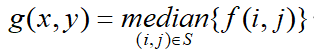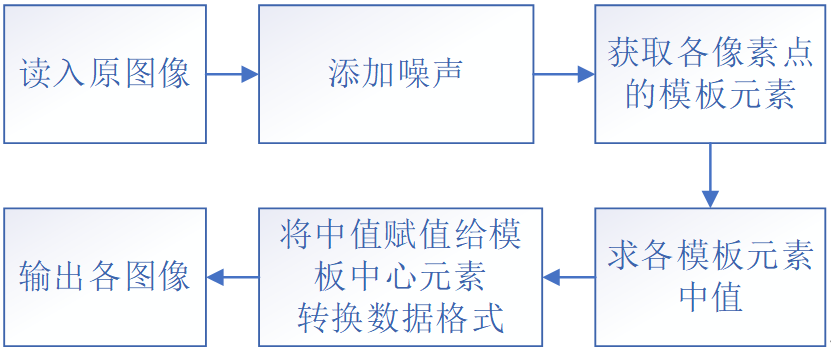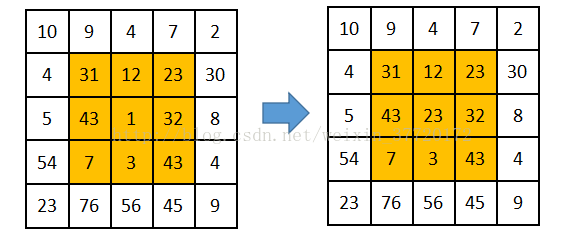• 2021-12-15 09:53:07

引言：在处理图像时，线性滤波将破坏边缘，而且不能有效滤除脉冲噪声。非线性滤波基于对输入信号序列的一种非线性映射关系，常可把某一特定的噪声近似映射为零而保留信号的重要特征，因而可以在一定程度上克服线性滤波的不足。浅谈中值滤波

## 中值滤波

1.原理
设有一个序列：x1，x2，x3，x4，x5，将它们按照绝对值大小重新排列此序列x3，x5，x2，x4、x1,重排以后的中值是×2，此值就作为滤波的输出。

2.Matlab代码实现

function g = median_filter(f)
[height, width]= size(f); % 输入图像的大小
g = zeros(height, width); % 为输出图像分配内存
for y = 1:height
for x = 1:width
x1 = x - 2; % 5x5 窗口的左列
x2 = x + 2; % 5x5 窗口的右列
y1 = y - 2; % 5x5窗口的顶行
y2 = y + 2; % 5x5窗口的底行
% 当邻域超出图像边界时，舍去超过边界的领域
x1 = max(x1, 1);
x2 = min(x2, width);
y1 = max(y1, 1);
y2 = min(y2, height);
window = f(y1:y2, x1:x2); % 5 x 5窗口
window = window(:); % 将窗口重新排列为列向量
g(y, x) = median(window); % 输出像素=窗口的中值
end
end
g = uint8(g); imshow(g); % 转换为8位图像并显示



## 中心加权中值滤波(the center weighted median filter)

1.原理
先解释一下加权中值滤波:
设有三个数（3，3，9），中位数为3，若加权系数为（1，1，3），即X3的加权系数W3=3，X1和X2的加权系数分别为W1和W2=1，也就是相当于X1重复1次，X2重复1次，X3重复3次，即相当于有5个数（3，3，9，9，9），中位数为9。一般中值滤波则相当于权系数为（1，1，1）。
而中心加权中值滤波:
就是对中心值加权（重复）
设有三个数（3，6，9），中位数为6，若中心加权系数为3，即X2的权系数W2=3，也就是相当于X2重复2次即相当于有5个数（3，6，6，6，9），中位数为6。一般中值滤波则相当于权系数为（1，1，1）。
2.Matlab代码实现

function g = cw_median_filter(f, M)
[height, width]= size(f);
g = zeros(height, width);
for y = 1:height
for x = 1:width
x1 = x - 2;
x2 = x + 2;
y1 = y - 2;
y2 = y + 2;
x1 = max(x1, 1);
x2 = min(x2, width);
y1 = max(y1, 1);
y2 = min(y2, height);
window = f(y1:y2, x1:x2);
window = window(:);
window1=[window;repmat(f(y,x),M-1,1)];  %M-1的原因是，window本身就包含了f(y,x) ,当M=1时就是中值滤波
g(y, x) = median(window1);
end
end
g = uint8(g);


matlab中repmat函数的用法matlab 计算机视觉
更多相关内容
• 数字图像处理，用C++语言，自己编写了中值滤波器以及均值滤波器，并和opencv自带函数对比，可直接运行。
• 编程实现灰度图像中值滤波平滑处理。滤波模板的大小自定（可为3×3、5×5、7×7、15×15等）。实验图像可从提供的实验图像集中的噪声图像中选取。 思考题：（选做） 编程实现灰度图像的均值滤波平滑处理；也可尝试...
• 设加噪图像为 f(x,y) ，经中值滤波处理后的图像为g(x,y) ，则： 式中，S是(x,y)像素点的邻域。本实验分别选用3×3、5×5、7×7的中值滤波窗口对图像进行处理。 需要注意的是，当模板滑动到图像边缘时，模板的部分行...

## 一、原理_中值滤波

中值滤波的基本思想是将图像中每个像素的灰度值用其邻域内像素灰度的中值代替，它是一种非线性平滑滤波算法。
设加噪图像为 f(x,y) ，经中值滤波处理后的图像为g(x,y) ，则：式中，S是(x,y)像素点的邻域。本实验分别选用3×3、5×5、7×7的中值滤波窗口对图像进行处理。

需要注意的是，当模板滑动到图像边缘时，模板的部分行或列就会处于图像之外，本实验可采用下面的任一种方法处理这种问题：
①先在图像边缘以外补上一行和一列灰度为零的像素点，再对原图像中的每个像素点进行模板处理。
②保留图像的边缘像素，对其它像素进行模板处理。

## 二、步骤

(1)读入原图像lena.bmp并显示；
(2)对原图像分别添加高斯噪声和椒盐噪声，并显示加噪图像；
(3)采用中值滤波进行去噪处理，并显示去噪图像；

## 三、实验图像lena.bmp

## 四、框图## 五、代码

%------------------------------------------------------------------------
% File name:           second_2
% Author:              Jasmine
% Descriptions:        中值滤波
%------------------------------------------------------------------------

%清空工作区
clc,clear,close all;
%读入原图像
%显示原图像
subplot(3,3,1);imshow(lena);title('原图');
%添加高斯噪声并显示
guassian_lena = imnoise(lena, 'gaussian', 0, 0.02);
subplot(3,3,2);imshow(guassian_lena);title('加入 均值：0 方差：0.02 的高斯噪声');
%添加椒盐噪声并显示
salt_lena=imnoise(lena,'salt & pepper',0.02);
subplot(3,3,3),imshow(salt_lena),title('加入噪声密度：0.02的椒盐噪声');
%高斯噪声   中值滤波
guassian_lena=rgb2gray(guassian_lena);
%%%%%%%%%%%%%%%%%%%%%%%%%%%%%%%%%%%%%%%%%%%%%%%%%%%%%%%%%%%%%%%%%%%%%%%%%%%
%手动中值滤波
n=3;    %模板大小
[height, width]=size(guassian_lena);   %获取图像的尺寸（n小于图片的宽高）
x1=double(guassian_lena);  %数据类型转换
x2 = x1;  %转换后的数据赋给x2
for i=1:height-n+1
for j=1:width-n+1
c=x1(i:i+(n-1),j:j+(n-1)); %在x1中从头取模板大小的块赋给c
e=c(1,:);      %e中存放是c矩阵的第一行
for u=2:n  %将c中的其他行元素取出来接在e后使e为一个行矩阵
e=[e,c(u,:)];
end
med=median(e);      %取一行的中值
x2(i+(n-1)/2,j+(n-1)/2)=med;   %将模板各元素的均值赋给模板中心位置的元素
end
end
result=uint8(x2);  %转换格式
subplot(3,3,4);imshow(result);title('高斯噪声 3*3中值滤波');
%5*5
%%%%%%%%%%%%%%%%%%%%%%%%%%%%%%%%%%%%%%%%%%%%%%%%%%%%%%%%%%%%%%%%%%%%%%%%%%%
%借助MATLAB中值滤波函数中值滤波（以下皆是）
gua_k2=medfilt2(guassian_lena,[5,5]);
subplot(3,3,5);imshow(gua_k2);title('高斯噪声 5*5中值滤波');
%7*7
gua_k3=medfilt2(guassian_lena,[7,7]);
subplot(3,3,6);imshow(gua_k3);title('高斯噪声 7*7中值滤波');

%椒盐噪声    中值滤波
salt_lena=rgb2gray(salt_lena);
%3*3
salt_k1=medfilt2(salt_lena);
subplot(3,3,7);imshow(salt_k1);title('椒盐噪声 3*3中值滤波');
%5*5
salt_k2=medfilt2(salt_lena,[5,5]);
subplot(3,3,8);imshow(salt_k2);title('椒盐噪声 5*5中值滤波');
%7*7
salt_k3=medfilt2(salt_lena,[7,7]);
subplot(3,3,9);imshow(salt_k3);title('椒盐噪声 7*7中值滤波');


## 六、运行结果中值滤波运行结果
展开全文matlab
• 实现了数字图像处理中的中值滤波，包含线、十字、X，方形、棱形、圆形窗口的滤波窗口。全都是自己写的，有注释。适用于交作业和研究学习中值滤波的朋友
• 基于MATLAB图像处理中值滤波、均值滤波以及高斯滤波的实现与对比: a) 中值滤波法是一种非线性平滑技术，它将每一像素点的灰度值设置为该点某邻域窗口内的所有像素点灰度值的中值. b) 均值滤波是典型的线性滤波算法...
• 111行C语言代码实现图像中值滤波，注释详细 以下凑字数 for(w=0;w;w++) { for(l=0;l;l++) { fputc(result[w][l],fq); fputc(result[w][l],fq); fputc(result[w][l],fq); } } ...
• 题目均值滤波和中值滤波 在自己的证件照中加入椒盐噪声高斯白噪声 分别用3*35*57*7 的均值滤波器和中值滤波器进行滤波 处理过程 1. 用imnoise函数在图像中分别加入椒盐噪声和高斯白噪声 2. 均值滤波用fspecial函数...
• ## MATLAB--数字图像处理中值滤波

万次阅读 多人点赞 2019-09-14 16:23:46
中值滤波是基于排序统计理论的一种能有效抑制噪声的非线性信号处理技术，中值滤波的基本原理是把数字图像或数字序列中一点的值用该点的一个邻域中各点值的中值代替，让周围的像素值接近的真实值，从而消除孤立的噪声...

## 中值滤波

概念
中值滤波是基于排序统计理论的一种能有效抑制噪声的非线性信号处理技术，中值滤波的基本原理是把数字图像或数字序列中一点的值用该点的一个邻域中各点值的中值代替，让周围的像素值接近的真实值，从而消除孤立的噪声点。方法是用某种结构的二维滑动模板，将板内像素按照像素值的大小进行排序，生成单调上升（或下降）的为二维数据序列。二维中值滤波输出为g（x,y）=med{f(x-k,y-l),(k,l∈W)} ，其中，f(x,y)，g(x,y)分别为原始图像和处理后图像。W为二维模板，通常为33，55区域，也可以是不同的的形状，如线状，圆形，十字形，圆环形等。

原理解释
其实中值滤波比较好理解，首先需要我们找到中值，再用其去替代一个位置的像素。比如在一副图像中，我们取3*3的模板，在这个模板中，一个有9个元素，我们找出其中的中值，用它去代替最中间的那个位置的像素，这就是中值滤波。
图示：## MATLAB实现

方法一：medfilt2()函数实现

 t=imread('a1.jpg');
t=rgb2gray(t);
t1=imnoise(t,'salt & pepper',0.3);
subplot(1,2,1),imshow(t1),title('加入椒盐噪声后')
t2=medfilt2(t1,[3 3]);
subplot(1,2,2),imshow(t2),title('中值滤波后')


效果图：注意：medfilt2()函数中的第一个参数必须是二维的，这就是为什么先要把图像灰度化的原因，那么有什么方法对彩色图像中值滤波呢？肯定可以的啊，分别对三个通道中值滤波就行了。

t=imread('a1.jpg');
t1=imnoise(t,'salt & pepper',0.3);
subplot(1,2,1),imshow(t1),title('加入椒盐噪声后')
t2=t
t2(:,:,1)=medfilt2(t1(:,:,1),[3 3]);
t2(:,:,2)=medfilt2(t1(:,:,2),[3 3]);
t2(:,:,3)=medfilt2(t1(:,:,3),[3 3]);
subplot(1,2,2),imshow(t2),title('中值滤波后')


效果图：方法二：自己编写中值滤波函数
中值滤波函数：

function [ img ] = median_filter( image, m )
%----------------------------------------------
%中值滤波
%输入：
%image：原图
%m：模板的大小3*3的模板，m=3

%输出：
%img：中值滤波处理后的图像
%----------------------------------------------
n = m;
[ height, width ] = size(image);
x1 = double(image);
x2 = x1;
for i = 1: height-n+1
for j = 1:width-n+1
mb = x1( i:(i+n-1),  j:(j+n-1) );%获取图像中n*n的矩阵
mb = mb(:);%将mb变成向量化，变成一个列向量
mm = median(mb);%取中间值
x2( i+(n-1)/2,  j+(n-1)/2 ) = mm;

end
end

img = uint8(x2);
end


主函数：

t=imread('a1.jpg');
t1=imnoise(t,'salt & pepper',0.3);
imshow(t1),title('加入椒盐噪声');
t2=median_filter(t,3);%调用函数
figure,imshow(t),title('中值滤波后')


效果图：## 更多

获取更多资料、代码，微信公众号：海轰Pro
回复 海轰 即可

展开全文MATLAB
• 数字图像处理 中值滤波 C语言 数字图像处理 中值滤波 C语言
• %% 中值滤波： I=rgb2gray(I); J=imnoise(I,'salt & pepper'); subplot(231),imshow(I);title('原图像'); subplot(232),imshow(J);title('添加椒盐噪声图像'); k1=medfilt2(J); %进行3*3模板
clear all;close all;
J=imnoise(I,'salt & pepper');

%% 中值滤波：
I=rgb2gray(I);
J=imnoise(I,'salt & pepper');
subplot(231),imshow(I);title('原图像');
subplot(232),imshow(J);title('添加椒盐噪声图像');
k1=medfilt2(J);               %进行3*3模板中值滤波
k2=medfilt2(J,[5,5]);       %进行5*5模板中值滤波
k3=medfilt2(J,[7,7]);       %进行7*7模板中值滤波
k4=medfilt2(J,[9,9]);       %进行9*9模板中值滤波
subplot(233),imshow(k1);title('3*3模板中值滤波');
subplot(234),imshow(k2);title('5*5模板中值滤波 ');
subplot(235),imshow(k3);title('7*7模 板中值滤波');
subplot(236),imshow(k4);title('9*9 模板中值滤波');
%输出信噪比：
s1=SNR1(J,k1)

%% 均值滤波
f=rgb2gray(f);
fn=imnoise(f,'salt & pepper');%用函数imnosie产生椒盐噪声
gm=medfilt2(fn);%中值滤波
figure;
subplot(131); imshow(f)
subplot(132); imshow(fn)
subplot(133); imshow(gm)
%信噪比：
s2=SNR1(fn,gm)

% muban_size = 3;%模板尺寸
% expand_size = floor(muban_size / 2);%扩展尺寸
% muban = 1 / (muban_size * muban_size) .* ones(muban_size, muban_size);
% expand_J = double(wextend('2D','zpd', J, expand_size));%扩展0，转double为了矩阵运算
%
% for i=1:M
%     for j=1:N
%         ave = sum( sum( expand_J(i:i+muban_size-1,j:j+muban_size-1) .* muban));
%取出扩展元素与模板相乘,并求矩阵元素之和
%         K(i,j) = ave;
%     end
% end
%
% K = uint8(K);%转int8，图像
%



信噪比子程序：

function snr = SNR1(I,In)
Ps=sum(sum(I.^2));
Pn=sum(sum((In-I).^2));
snr=10*log10(Ps/Pn);
end

展开全文matlab 均值算法
• 数字图像处理中值滤波MATLAB程序 ...... ...... k=floor(3*3/2)+1; [M,N]=size(a1); uint8 Y=zeros(M,N); funBox=zeros(3,3); temp=zeros(9); ......
• 一个简单的数字图像处理软件，实现了均值、最大值、最小值、直方图均衡、中值滤波、Sobel锐化、DCT变换和IDCT还原以及图像旋转等基本功能。
• 文章目录一、实验任务与内容二、实验原理2.1 均值滤波2.2 中值滤波三、编程思路四、效果展示五、源代码Filter.hFilter.cppmain.cpp 一、实验任务与内容 利用VC++编写一个3*3的均值滤波或中值滤波程序 （1）采用参数...算法 c++
• 中值滤波作为图像处理中的一种非线性滤波技术，在有效抑制脉冲噪声的同时能很好地保护图像信号的细节信息，尤其是在处理椒盐噪声方面效果较好，得到了广泛的研究和应用。文章通过对中值滤波及其改进方法的研究，比较...
• 中值滤波的原理就是用一个含有奇数点的滑动窗口，将窗口正中那点的值用窗口内各点的中值代替。设计中只考虑方形这一种情况的中值滤波，采用的中值滤波的窗口为3×3的矩形窗口，共9个像素。实验结果表明通过中值滤波...
• 中值滤波器是非线性滤波器的一个例子，它在保留图像特征方面非常有效。 但是，滤波器的窗口大小直接影响中值滤波器的性能。 较小的窗口保留了特征，但会导致噪声抑制的减少。 在较大窗口的情况下，噪声抑制很高，但...算法
• 基于Matlab与QuartusII的数字图像中值滤波仿真，张贝，焦淑红，本文提出了一种基于Matlab与QuartusII的在FPGA中导入测试图像数据的方法，并进行了对图像中值滤波的处理，最后通过Matlab软件对仿真数据��Matlab
• VC平台，绝对能运行。带图片，利用描点方式，先将jpg文件解压成bmp文件。利用中值滤波都方法将原图滤波后显示，逐点描图，便于初学者学习。
• 基于MATLAB GUI实现数字图像中值滤波.pdf
• VC++数字图像处理 中值滤波，基于VC++的数字图像处理实现中值滤波
•计算机视觉 opencv
• 然后分别进行均值滤波和中值滤波，并把处理后的图片保存。2. 图像频域处理，对图像进行傅里叶变换，进行高通滤波，显示处理结果。3. 图像平移和旋转4. 分别用OSTU阈值法和全局阈值法分割图像，然后进行腐蚀和膨胀...
• } } } } /* * description:对二维矩阵进行中值滤波，使用模板运算 * paramaters: * matrix是矩阵索引 * width：二维矩阵的宽 * hidth：二维矩阵的高 * target:保存修改过之后的临时数组 * return:整个数组的参数 * ...矩阵
•matlab 计算机视觉
• 对lena.bmp图像分别做33、55、77、99的中值滤波，并分析模板的大小对图像质量的影响。 %中值滤波 I=imread('lena.bmp'); J=imnoise(I,'salt & pepper',0.02); figure subplot(231),imshow(I);title('原图') ...计算机视觉 opencv
• CV小白从0开始学数字图像处理 10中值滤波（Median filter） 使用中值滤波器（3x 3大小）进行降噪处理 中值滤波器是一种可以使图像平滑的滤波器。这种滤波器用滤波器范围内（在这里是3x3）像素点的中值进行滤波，在...python 计算机视觉 opencv 人工智能
• ## 图像处理：中值滤波&均值滤波

万次阅读 多人点赞 2018-08-16 10:57:19
转自：openCV之中值滤波&amp;均值滤波（及代码实现）：https://blog.csdn.net/weixin_37720172/article/details/72627543 ...图像滤波，即在尽量保留图像细节特征的条件下对目标图像的噪声进行抑...均值滤波...